# Modeling and Control of Electric Motors U.S. Electric Motors Type Dripproff 1150 RPM/10 HP/240 Volt Using MATLAB/Simulink

Modeling and Control of Electric Motors U.S. Electric Motors Type Dripproff 1150 RPM/10 HP/240 Volt Using MATLAB/Simulink
Department of Electrical Engineering, Faculty of Engineering, Universitas Musamus, Merauke 99611, Indonesia

Corresponding Author Email:
frederik@unmus.ac.id
Page:
223-232
|
DOI:
https://doi.org/10.18280/ejee.220303
6 January 2020
|
Accepted:
13 March 2020
|
Published:
30 June 2020
| Citation

OPEN ACCESS

Abstract:

Identification motor DC HP large by using modeling, control through the computer program simulation will help a lot in observing the dynamic characteristics of the motor. The resulting information is useful in planning and investment costs. Besides, with the computer program is able to do the simulation process interference on the system without having to risk the safety of the system. In making DC motor model the method used is mathematical modeling method, box chart and Simulink/MATLAB. While the control system applied is open-loop control through setting the armature voltage and series resistance and closed loop with algorithmic controller. The modeled motor is motor DC U.S. Electric Motors Type Dripproff 1150 RPM/10 HP/240 Volt.

Keywords:

simulation process interference DC motor model, box chart and Simulink/ MATLAB

1. Introduction

Motor DC (Direct Current) or direct current motors are included in the category of motor type most widely used both in industrial environments, household appliances to children's toys or as instruments supporting the electronic instrument system. DC motors have a variety of types ranging from the type of permanent magnet, series, shunt or type of compound magnet. The type of DC motor is implemented based on the type of magnet used .

DC motors are widely used, because they have these good performances, such as good starting and speed regulation, wide speed range, smooth load, strong overload capability, little electromagnetic interference and low maintenance, so it is necessary to study the speed control of the DC motor .

The speed of a DC motor can be adjusted over a wide range according to three magnetization sources, separately excited field, self-excited field or permanent field. A self-excited field motor is controlled by either the variable filed voltage or by changing the excitation resistance value. As the control requirements in industrial applications are gradually improved, the accurate simulation of the speed regulation system is particularly demanded, it permits the mathematical analysis by combining the characteristics of DC motor with the model- based control strategies, so as to overcome the traditional shortcomings like unstable speed and torque ripple, etc., thus to enhance the speed control efficiency of this complex, non- linear and strong coupling system .

The development of mathematical and physical Modeling based simulators in the Simulink, as interactive tools to demonstrate the DC motor responses in terms of current, speed and torque. This type of Modeling requires to join the physical components with physical connections to define the underlying dynamic equations of the DC motor. This approach is further compared with the analytical model in Simulink. A comparative study of the model for the separately excited Dc motor has shown that the models have their own merits and demerits .

The block diagram of a DC motor is developed and by using SIMULINK, the block diagram is simulated, for simulation of motor, some data is also required like torque constant which is obtained by experimental investigations. By varying certain parameters of the DC motor Simulink block, the output waveform of the simulation would change accordingly. These parameters include the field current, armature circuit resistance and armature voltage. The simulation and modelling of the DC motor also gave an inside look of the expected output when testing the actual DC motor [5, 6].

The main purpose of this paper is to produce DC motors capacity of 1150 RPM/ 10 HP/ 240 Volt, through mathematical modeling simulations, box charts, Simulink / MATLAB and controlling with open loop control through setting the resistance armature voltage and series resistance and closed cycle with an algorithm controller.

2. Direct Current C Motors

2.1 DC motor characteristics

The general operational characteristics common to all DC motors are shown in Figure 1.

Most electric motors exhibit characteristics similar to those shown in the block diagram. The amount of mechanical load applied to the shaft of a motor determines its operational characteristics. As the mechanical load is increased, the speed of a motor tends to decrease. As speed decreases, the voltage induced into the conductors of the motor through generator action (cemf) decreases. The generated voltage or counterelectromotive force depends upon the number of rotating conductors and the speed of rotation. Therefore, as speed of rotation decreases, so does the cemf.

## 1.pngFigure 1. Operational characteristics of DC motors

Since the cemf is in opposition to the supply voltage, the actual working voltage of a motor will increase as the cemf decreases. As the result of an increase in working voltage, more current will flow through the armature conductors that are connected to the DC power supply. Since torque is directly proportional to armature current, the torque will increase as the armature current increases. Since torque is directly proportional to armature current, the torque will increase as the armature current increases. Maximum armature current flows when there is no cemf. As cemf increases, armature current decreases. Thus, resistances in series with the armature circuit are often used to compensate for the lack of cemf, and to reduce the starting current of a motor. After a motor has reached full speed, these resistances may be bypassed by automatic or manual switching systems, in order to allow the motor to produce maximum torque .

In determining the functional characteristics of a motor, the torque developed can be expressed as:

T=K Φ IA  (1)

where:

T= the torque in foot-pounds,

K= a constant based on physical characteristics (conductor size, frame size, etc.)

Φ= the quantity of magnetic flux between poles, and

IA= the armature current in amperes.

2.2 Principle of operation

The principle of operation on a DC motor as shown in Figure 2 requires a DC voltage source. When the two ends of the coil are connected across a DC voltage source, it will cause current, I, to flow through it. Hence, a force is exerted on the coil as a result of the interaction of magnetic field and electric current. The force on the two sides of the coil is such that the coil starts to move in the direction of force .

## 2.png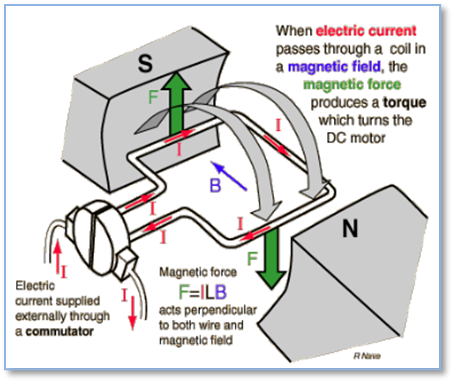Figure 2. Torque production in a DC motor

When an electric current pass through a coil that is in an electric field, then the magnetic force will produce a torque that will rotate the DC motor, as illustrated in Figure 2. When the coil spins on the magnetic field, a voltage is raised across the edges. This voltage is the back emf, which is proportional to the rotational speed of the coil and opposite to the current flow, as follows:

eb=K. $\Phi$. $\omega$m   (2)

with:

eb= emf back (volts),

$\omega_{m}$ = rotational speed of the coil (rad.sec).

Eqns. (1) and (2) form the basis of the working of DC motors.

2.3 Mathematical model of DC motor

The DC motor has two main components. The first is an electrical component consisting of resistance R, inductance L, input voltage V, and back-electromotive force E. The second is the mechanical component, which creates a mechanical rotational motion on the shaft. It means the moment of inertia of the actuator and the load inertia J and damping b. The circuit diagram of the DC motor is shown in Figure 3 .

## 3.png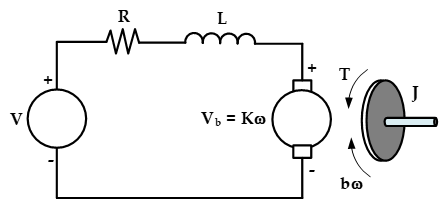Figure 3. Schematic representation of the DC motor based 

Explanation of working principle of DC motor can be made motor mathematic model, which consists of electric models and dynamic models, as follows :

(1) Physical model

## 4.png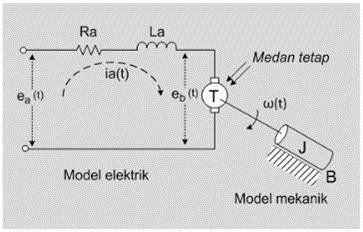Figure 4. Physical model of DC motor

(2) Dynamic model

The physical model in Figure , then the dynamic model of DC motor is:

$\frac{d i_{a}(t)}{d t}=\frac{1}{L a}\left[e_{a}(t)-e_{b}(t)-R a i_{a}(t)\right]$  (3)

$\frac{d i_{a}(t)}{d t}=\frac{1}{J}\left[T(t)-B_{\omega}(t)\right]$  (4)

T(t)=K m ia (t)  (5)

Eb(t)=K b $\omega$(t)  (6)

where, the equations are an explanation:

1. Eq. (3) shows the electrical properties
2. Eq. (4) indicates mechanical properties
3. Eq. (5) shows the motor properties
4. Eq. (6) shows the nature of the generator

The value of the DC motor parameters to be used in this simulation is shown in Table 1.

Table 1. DC motor data *)

 NO DATA SYMBOL VALUE UNIT 1 Mechanical power *) P 10.00 HP 7460.00 Watt 2 Speed of play *) $\omega$ 1150.00 RPM 120.43 Rad/sec 3 Anchors Voltage *) Ea 240.00 Volt 4 Flow Anchor *) Ia 35.00 Ampere 5 Prisoner Anchors *) Ra 0.33 Ohm 6 Momen Inersia*) J 3.40 Lb ft2 0.14 N.m.sec2/rad 7 Inductance anchors *) La 0.009 Henry 8 Torsi**) T 61.95 N.m 9 Motor Constant **) Km 1.7699 N.m/A 10 Generator constants **) Kb 1.8970 Volt.sec/rad 11 load**) B 0.5144 N.m.sec/rad
*) Fabrication data (attachment), **) The calculated data

## 5.png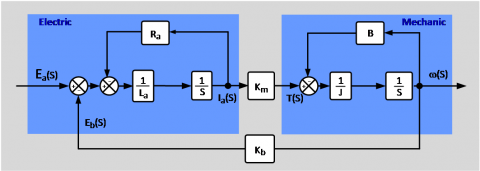Figure 5. Chart box model DC motor

## 6.png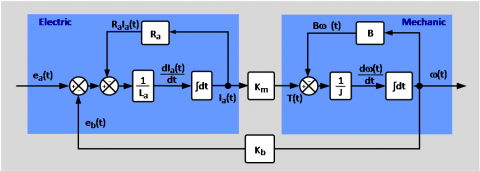Figure 6. Box model of a DC motor using the Laplace transform concept

## 7.png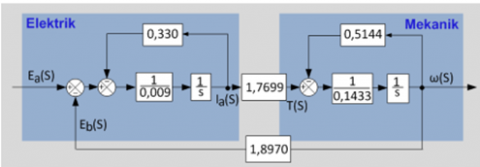Figure 7. Model chart model DC motor controlled armature

3. Modeling Controlled Armature DC Motor

Modeling controlled armature DC motor, then first made a model of a square chart based on electrical models and models dynamic.

3.1 Box chart model

Completion with Laplace transform then box diagram in Figure 5 above can be modeled, as follows:

Completion by replacing the existing values in Table 1, then generated controlled DC motor model armature, as shown in Figure 7 above.

Furthermore, to find out the performance analysis of the armature controlled model system, it is necessary to know the transfer function of the model.

3.2 Transfer function

Transfer functions are defined as a comparison between Laplace transforms the output of the input Laplace transform assumes all initial conditions are zero. Transfer function which will be determined .

(1) Enter the armature voltage (Ea), the output of the rotational speed ω.

## 8.png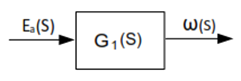Figure 8. Box chart transfer function for rotating speed ω

By using the algebraic rules in the box diagram of Figure 8 above, the above can be simplified as follows:

## 9.png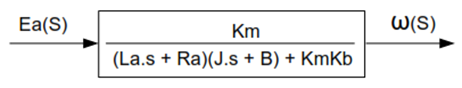Figure 9. Box chart algebraic transfer function for rotating speed ω

Then the ratio of the deviations from the system in Figure 9 above is:

\begin{aligned} \mathrm{G}_{1}(\mathrm{s})=& \frac{\omega \cdot(s)}{E_{a}(s)}=\frac{K_{m}}{\left(L_{a} S+\cdot R_{a}\right)(J s+B)+\cdot K_{m} K_{b}} \\ &=\frac{K_{m}}{\frac{L_{a} \cdot J}{s^{2} \frac{R_{a} \cdot J+\cdot B L_{a}}{L_{a} \cdot J} s+\frac{\left(B R_{a}+\cdot K_{m} K_{b}\right)}{L_{a} J}}} \\=& \frac{K_{o}}{S^{2}+2 \zeta \omega_{n} s+\cdot \omega_{n}^{2}} \end{aligned}  (7)

with:

ζ =damping ratio

ωn=Natural frequency is not muffled (undamped natural frequency, [rad/sec])

(2) Input armature voltage (Ea), output of a current armature (Ia), as shown in Figure 10.

$\mathrm{G}_{1}(\mathrm{s})=\frac{I_{a}(s)}{E_{a}(s)}=\frac{I_{a}(s)}{E_{a}(s)} \chi \frac{\omega(s)}{E_{a}(s)}$  (8)

## 10.png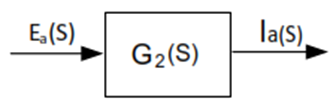Figure 10 . Box chart transfer function for output of a current armature (Ia)

From Figure 6 above, it shows that:

\begin{aligned} \frac{\omega(s)}{I_{a}(s)} &=\frac{K_{m}}{J s+B} \rightarrow \frac{I_{a}(s)}{\omega(s)}=\frac{J s+B}{K_{m}} \\ \mathrm{G}_{2}(\mathrm{S})=& \frac{I_{a}(s)}{\omega(s)} x \frac{\omega(s)}{E_{a}(s)} \\ &=\frac{J s+B}{K_{m}} x \frac{K m}{\left(L_{a} s+R_{a}\right)(J s+B)+K m K b} \\ &=\frac{\frac{1}{L a} s+\frac{B}{L a J}}{S^{2}+\frac{\left(R_{a} J+B L_{a}\right)}{L_{a} J} s+\frac{\left(B R_{a}+K m K b\right)}{L_{a} J}} \\ &=\frac{a s+b}{S^{2}+2 \zeta \omega_{n} s+\omega_{n}^{2}} \end{aligned}  (9)

3.3 The analytical solution of the rotational speed ω(t) and the armature current ia(t) of the DC motor modeling

Solution analytically needs to be done in order to verify the model made whether it really is in accordance with the real conditions that exist. The result of the resulting analytical solution will be compared with the technical data (fabrication) of the DC motor. If the result is the same or has a small error, then the built model is acceptable. If the result does not match or has a big error, then the built model is unacceptable.

3.3.1 Analytical solution for rotational speed ω(t)

The analytical solution for obtaining ω (t) is generated from the inverse transform of Laplace Eq. (5) and data from Table 1, as follows:

\begin{aligned} \omega(\mathrm{s}) &=\frac{K_{o}}{S^{2}+2 \zeta \omega_{n} s+\omega_{n}^{2}} x E_{a}(\mathrm{s}) \\ \omega(\mathrm{t}) &=\mathcal{L}^{-1}[\omega(s)] \\ &=\mathcal{L}^{-1}\left[\frac{K_{0} E_{a} n o m}{S\left(S^{2}+2 \zeta \omega_{n} S+\omega_{n}^{2}\right)}\right] \\ &=K_{0} E_{a} n o m\left[\frac{1}{\omega_{n}^{2}}-\frac{1}{\omega_{n} \omega_{d}} e^{-\zeta \omega_{n} t} \sin \left(\omega_{d} t+\phi\right)\right] \end{aligned}

t ≥ 0  (10)

with,

ωd= natural frequency damped

ωd= ωn $\sqrt{\left(1-\zeta^{2}\right)}$

$\phi=\cos ^{-1}(\zeta)$

3.3.2 Analytical solution for current armature ia(t)

The analytical solution for obtaining it (t) is generated from the inverse transform of Laplace equation 6 and a data from Table 1, as follows:

\begin{aligned} I_{a}(s) &=\frac{a s+b}{s^{2}+2 \zeta \omega_{n} S+\omega_{n}^{2}} x E_{a}(S) \\ i_{a}(t) &=\mathcal{L}^{-1}\left[I_{a}(s)\right] \\ &=\mathcal{L}^{-1}\left[\frac{a \cdot E_{a} n o m}{\left(S^{2}+2 \zeta \omega_{n} S+\omega_{n}^{2}\right)}+\frac{b \cdot E_{a} n o m}{S\left(S^{2}+2 \zeta \omega_{n} S+\omega_{n}^{2}\right)}\right] \\ &=\frac{a \cdot E_{a} \operatorname{mom}}{\omega_{d}} e^{-\zeta \omega_{n} t} \sin \omega_{d} t+\frac{b \cdot E_{a} n o m}{\omega_{d}}-\\ \frac{a \cdot E_{a}}{\omega_{n}} \operatorname{nom}_{d_{d}} e^{-\zeta \omega_{n} t} \sin \left(\omega_{d} t+\phi\right) &=K_{0} E_{a} \operatorname{nom}\left[\frac{1}{\omega_{n}^{2}}-\frac{1}{\omega_{n} \omega_{d}} e^{-\zeta \omega_{n} t} \sin \left(\omega_{d} t+\phi\right)\right] \\ t & \geq 0 \end{aligned}  (11)

3.4 Calculate value from a, b, Ko, ωn, ωd and $\phi$

Based on Eq. (5) and (6) and data in the table, then:

$\mathrm{a}=\frac{1}{L_{a}}=\frac{1}{0,0090}=111,111111$

$\mathrm{b}=\frac{B}{L_{a J}}=\frac{0,5144}{0,0090 \times 0,1433}=398,852446$

$\mathrm{K}_{\mathrm{a}}=\frac{K_{m}}{L_{a} l}=\frac{1,7699}{0,0090 \times 0,1433}=1372,334651$

$\omega_{n}^{2}=\frac{\left(B R_{a}+K_{m} K_{b}\right)}{L_{a} J}=2734,940141$

$\omega_{\mathrm{n}}=\sqrt{2734,940141}=52,296655 \mathrm{rad} / \mathrm{sec}$

$2 \zeta \omega_{n}=\frac{\left(R_{a} J+B L_{a}\right)}{L_{a} J}=40,256339$

$\zeta=\frac{40,256339}{2 \omega_{n}}=0,384884$

$\omega_{n}=\omega_{n} \sqrt{1-\zeta^{2}}=48,267970 \mathrm{rad} / \mathrm{sec}$

$\phi=\cos ^{-1}(\zeta)=1,175714 \mathrm{rad}$

3.5 Verify ω(t) and Ia(t)

3.5.1 Verify ω(t)

By entering the result data into Eq. (9) it is generated:

ω(t)=120,426883 – 130,478310 e-20,1201691. Sin (48, 267970.t +1,175714) rad/sec

Verify:

When t = 0

ω(t)= 120,426883 – 120,426883= 0 rad/sec

At time t = ∞, state steady-state

ω(∞) = 120,426883 – 0=120,426883 rad/sec

3.5.2 Verify Ia(t)

By entering the calculated data into the Eq. (10) then the result:

ia(t)=552,471268 e-20,128169.tsin 48,267970.t + 35,000615 – 37,921941.e-20,128169.t. sin (48,267970.t+1,175714) Ampere.

Verify:

When

t = 0,

ia(t)=35,000615 – 35,000615=0 ampere

ia(∞)   = 0 + 35,000615 – 0 = 35 ampere

From the verification result, the result of the analytical solution ω(t) and Ia(t) modeling with the fabrication data is the same. Thus the model built is correct. The following graphs present the exact solution ω(t) and Ia(t), as shown in Figure 11 and 12.

## 11.png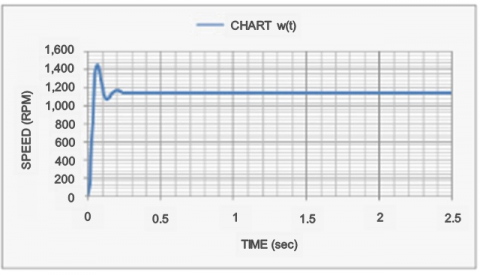Figure 11. Speed rotating ω(t)

## 12.png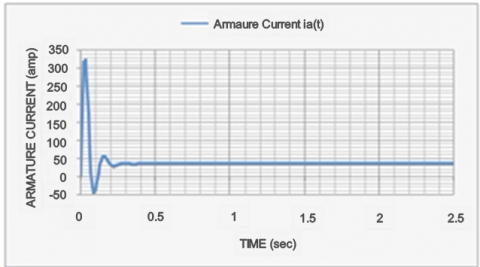Figure 12. Armature current Ia(t)

Based on controlled DC motorcycle box model model shown in Figure 7, then it can be built simulink model see Figure 9. By giving input voltage Ea=240 V, series resistance (Rs) 0 ohm and external load=0.9.B (N.m.sec/rad) the resulting graph of the exact solution ω(t) and ia(t), as follows.

When compared with analytical solutions, then the graph ω(t) and it(t) show similar results. This indicates that the Simulink model can also model the controlled DC motor armature.

4. Open Loop Control

A system whose output has no effect on control action is called an open-loop control system. In other words, the control system. The closed-loop output cannot be used as a feedback ratio with the input.

DC open-duty motor control can be done in two ways:

(1) Series arrest settings (Rs)

(2) Setting armature voltage (Ea)

The simulink model of controlling the open cycle DC motor controlled armature either by setting the series resistance or the armature voltage is as in Figure 14. The plant model of the DC motor is the same as the simulink model shown in Figure 13 below.

4.1 Series resistance settings

This method is done by adding a series resistance (Rs) connected with armature resistance (Ra), with the intention of increasing the armature resistance value so that there is no overshoot for the pace speed ω(t) and the armature current ia(t)

At the start of the series resistance (Rs) in the maximum set such that Ra’= Rs + Ra, where as time goes by the value of Rs will be reduced slowly until it reaches zero. On the contrary, the value of Rs is increased slowly so as to achieve its maximum value, when the motor is stopped.

How to set this Rs value in general using a small dc motor or using a resistor bank. The Rs array resistance settings used in the simulink model (as shown in Figure 14 below) is by slowly shifting the Rs value up to the maximum value within 2 sec. Move manual switch 1 at position 1, move manual switch at position 2 (motor given simulation in the form of interference on external load), and provide a series resistance value according to the desired damping characteristics:

(1) Underdamped when Rs < 0.620716 $\Omega$

(2) Critical damped when Rs = 0.620716 $\Omega$

(3) Overdamped when Rs > 0.620716 $\Omega$

Here is shown graph in Figure 15, about speed rotation ω(t) and armature current ia(t) generated at the time Rs =5 $\Omega$, or ζ=4,331968 Overdamped.

4.2 Voltage armature settings

This is done by raising the (Ea) armature voltage slowly until it reaches its nominal value, i.e. 240 volts within 2 sec, at start. Instead armature tilt is slowly lowered to 0 volts at the time of stop.

This arrangement also aims to reduce the overshoot for the pace speed ω (t) and the armature current he (t). Move the manual switch 1 in position 2, move the manual switch at position 2 (the motor is given simulation in the form of interference on the outside load). The value of the series resistance Rs = 0 ohms. The following graph shows in Figure 16 about the rotational speed ω(t) and the armature current ia(t).

5. Closed Loop Control

A system that has an effect on the control action is called a closed-loop control system. In other words, the closed-loop control system is used as a feedback comparison with the input. Therefore closed-loop control systems are often referred to as feedback control systems.

In a closed-loop control system, the error signal works, i.e.; error = setpoint - an output signal which is then passed to the controller in such a way as to reduce the error and bring the system output to the desired value(setpoint/reference). Thus, the term closed-loop control action always means the use of feedback control action to reduce errors.

## 13.png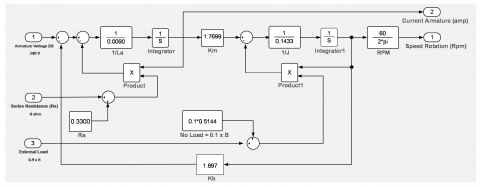Figure 13. Simulink model controlled DC motor armature

## 14.png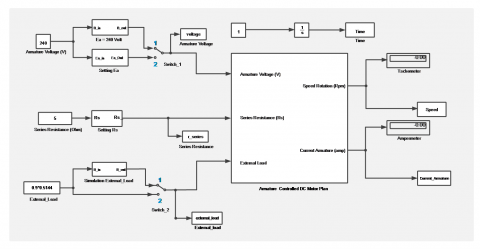Figure 14. Simulink model controlled DC motor armature open loop with setting the series resistance and armature voltage

## 15.png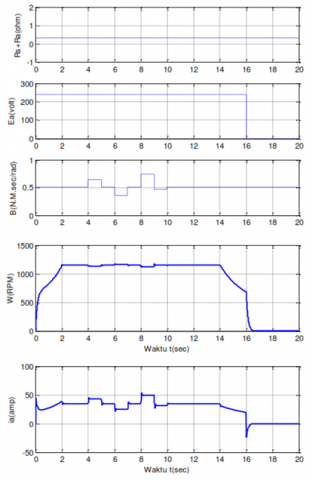Figure 15. Graphs Rs, Ea, B, w(t) and ia(t) for overdamped characteristics

## 16.pngFigure 16. Graphs Rs, Ea, B, w(t) and ia(t) for setting armature voltage

## 17.png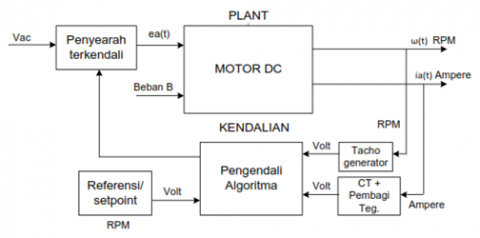Figure 17. Block diagram of control closed loop

5.1 Close loop modelling of a DC motor

The controlled DC motor plant consists of an input, an Ea voltage armature, Rs series resistance and external load B and two outputs, i.e., the rotational speed ω (t) and the armature current ia(t). Thus the control system used is in the form of algorithmic controller which integrates feedback of output signal of current armature and speed of play as shown in Figure 17.

To equate the signal output current and speed, then used the transducer:

(1) Current transformer (CT) equipped with a voltage divider, so that the output signal of the armature current ia(t) (ampere) is converted into a voltage signal (volt).

(2) Tachogenerator, which is an electromechanical device that converts mechanical energy into electrical energy. This device works as a voltage generator, with an output voltage proportional to the magnitude of the rotational speed of the motor rotor. Thus the output signal of the rotational speed ω (t) can be converted into a voltage signal (volt). The reference signal (setpoint) is converted into alpha signal) by using the MATLAB function Rpm_alpha as follows:

## 0.png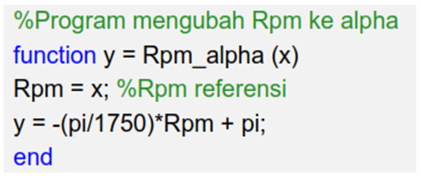5.1.1 Algorithm controller

Model simulink controller algorithm (Figure 18) is as follows:

## 18.png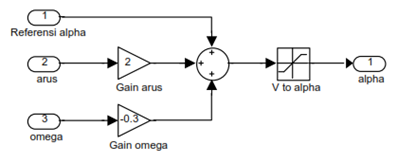Figure 18. Simulink model of the closed loop controller algorithm

In this algorithm controller the three input signals will be summed. The sum of this sum is limited from angle 0 sd 2Π rad. This alpha output signal will be forwarded to the rectifier controlled to control the amount of AC voltage to be rectified.

5.1.2 Controlled rectifier

A controlled rectifier is a converter device from AC voltage to DC voltage. The amount of voltage to be rectified is dependent of the alpha input signal of the controller. The simulink model of this device in Figure 19 is as follows:

## 19.png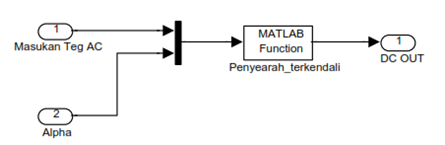Figure 19. Simulink model for rectifier

The work of a controlled rectifier is presented in the MATLAB function rectifier controlled as shown below.

The principle of this controlled rectifier device is based on the concept of a full-wave rectifier. Because one wave cycle (0 sd 2Π rad) consists of four parts, then the AC input voltage on the controller first divided in the quantity per wave section

If the alpha input of the controller is in the region 0 <alpha = Π rad, then the voltage magnitude to be rectified does not change in polarity, since cos (alpha) in quadrant 1 & 2 is positive.

## 01.png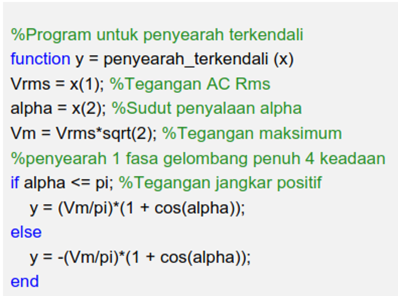y = -(Vm/pi)*(1+ cos(alpha)).

Whereas if the alpha angle is in the region Π <alpha <2Π, then the voltage magnitude will change the polarity. With the principle of full wave rectifier, then the polarity must be reversed so that the required polarity changes in the calculation of the voltage values are rectified.

y =  -(Vm/pi)*(1+ cos(alpha)).

The plant of controlled DC motor armature is the same as Figure 13. So, the simulink model of controlled closed cycle motor cycle controlled DC is as follows in Figure 20.

Given voltage Ea=240 volts, Rs=0 ohms, outside load B, and ω=1150 RPM, then the following graph presented from the simulink model results in Figure 21.

## 20.png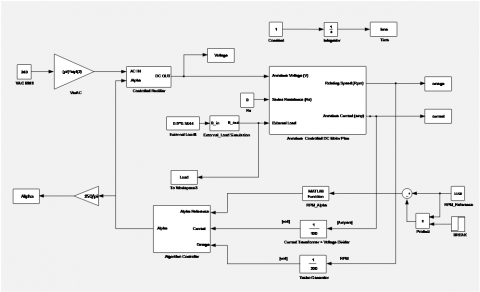Figure 20. Simulink model of closed loop controlled the armaturae control DC Motor

## 21.png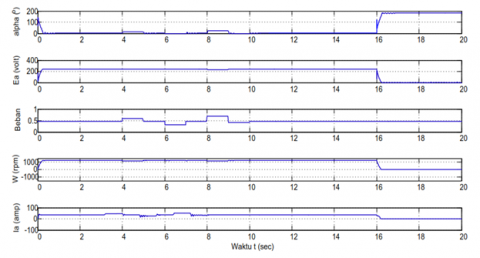Figure 21. Graph of alpha, Ea, Load, ω(t) and Ia(t) for closed loop control

6. Conclusion

Based on the above analysis and discussion it can be concluded as follows:

(1) Planning a control system (DC motor) will not be separated from an assumption how this system will work properly through an angle review behavior or system characteristics. The main characteristics to note are the electrical characteristics of the system such as start current spikes and rotational speed, transient voltage profile to transient analysis at the time of system interference.

(2) Mathematical models (electric and dynamic), box model models and simulink models in controlled DC motor control system design in this paper, show optimal results because the resulting system response has been in accordance with analytical solutions.

(3) An unmanageable controlled armature motor model produces the highest overshoot rotational velocity ω (t) and current armature (t) when compared to the open-loop control model and the closed-loop. While the closed cycle control model shows the results of ω (t) and it (t) is better than closed-loop control. This suggests that the system response of the uncontrolled model can be improved by the open-loop control model and can be rectified by the closed-loop control model.

(4) The characteristics of open-loop control systems are, 1) The control action is independent of the system output; 2) Cannot provide corrections / compensation in case of interference; 3) Simpler.

(5) The characteristics of a closed-loop control system are, 1) The control action depends on the output of the system; 2) Overcoming the weakness of the open-loop control system because it can provide corrections when there is interference; 3) There may be over correction, so the system becomes unstable; 4) More complex because of the more components.

References

 Boroujeni, M.S., Markadeh, G.A., Soltani, J. (2017). Torque ripple reduction of brushless DC motor based on adaptive input-output feedback linearization. ISA Transactions, 70: 502-511. https://doi.org/10.1016/j.isatra.2017.05.006.

 Tang, W.J., Cao, S.Y. (2018). A fast realization method of fuzzy PID control for DC motor. In 2018 37th Chinese Control Conference (CCC), pp. 5131-5135. https://doi.org/10.23919/ChiCC.2018.8483184.

 Hu, X., Ming, P.L., Wei, X.Z. (2017). Analysis and comparison of Various speed control strategies on the performance of DC Motor. In 2017 International Conference on Industrial Informatics-Computing Technology, Intelligent Technology, Industrial Information Integration (ICIICII), Wuhan, China, pp. 290-293. https://doi.org/10.1109/ICIICII.2017.90

 Okubanjo, A., Oyetola, O., Olaluwoye, O., Olufemi, A.L.A.O., Olateju, A., Abatan, T. (2019). Modeling and simulation of DC motor using simelectronics and simulink. Gazi Mühendislik Bilimleri Dergisi (GMBD), 5(1): 91-100. https://doi.org/10.30855/gmbd.2019.01.09

 Bandana, Gaur, S. (2017). Simulation of transient behaviour of Dc motor using MATLAB / simulink. International Journal of Advanced Research in Electrical, Electronics and Instrumentation Engineering, 6(2): 575-582. https://doi.org/10.15662/IJAREEIE.2017.0602012

 Hardiantono, D., Sumbung, F.H. (2016). Rancang bangun unit pembangkitan dan modul pengukurannya untuk pembangkit listrik tenaga hybrid (angin dan matahari). MUSTEK ANIM HA, 5(3): 231-245. https://doi.org/10.35724/mustek.v5i3.629

 Shertukde, H.M. (2019). Electrical Machines. Power Systems Analysis Illustrated with MATLAB® and ETAP®. First edition. | Boca Raton, FL : CRC Press/Taylor & Francis Group, 2018.: CRC Press. https://doi.org/10.1201/9780429436925-2

 Rudra, R., Banerjee, R. (2017). Modeling and simulation of DC motor speed regulation by field current control using MATLAB. International Journal of Computer and Electrical Engineering, 9(2): 502-512. https://doi.org/10.17706/IJCEE.2017.9.2.502-512

 Naung, Y., Anatolii, S., Lin, Y.H. (2019). Speed control of DC motor by using neural network parameter tuner for PI-controller. In 2019 IEEE Conference of Russian Young Researchers in Electrical and Electronic Engineering (EIConRus), pp. 2152-2156. https://doi.org/10.1109/EIConRus.2019.8656911

 Waroh, A.P. (2014). Analisa dan simulasi sistem pengendalian motor DC. Jurnal Ilmiah Sains, 14(2): 80-86. https://doi.org/10.35799/jis.14.2.2014.5935

 Tirono, M., Nayiroh, N. (2012). Pemodelan dan pembuatan simulasi kestabilan respon transien motor DC menggunakan graphical user interface (GUI) pada Matlab. Jurnal Neutrino: Jurnal Fisika dan Aplikasinya. https://doi.org/10.18860/neu.v0i0.1605